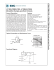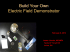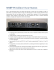# Lecture 20

## Transcription

Lecture 20
```Physics 2102
Jonathan Dowling
Lecture 20
Ch. 31: Electrical Oscillations, LC
Circuits, Alternating Current
What are we going to learn?
• Electric charge
 Electric force on other electric charges
 Electric field, and electric potential
• Moving electric charges : current
• Electronic circuit components: batteries, resistors, capacitors
• Electric currents  Magnetic field
 Magnetic force on moving charges
• Time-varying magnetic field  Electric Field
• More circuit components: inductors.
• Electromagnetic waves  light waves
• Geometrical Optics (light rays).
• Physical optics (light waves)
Oscillators in Physics
Oscillators are very useful in practical applications,
for instance, to keep time, or to focus energy in a
system.
All oscillators operate along the same
principle: they are systems that can store
energy in more than one way and
exchange it back and forth between the
different storage possibilities. For instance,
in pendulums (and swings) one exchanges energy
between kinetic and potential form.
In this course we have studied that coils and capacitors are devices
that can store electromagnetic energy.
energy In one case it is stored in a
magnetic field, in the other in an electric field.
A mechanical oscillator
Etot = Ekin + E pot
1
1
Etot = m v 2 + k x 2
2
2
dEtot
1
dv 1
dx
= 0 = m&\$ 2v #! + k &\$ 2 x #!
dt
2 % dt " 2 % dt "
dv
m +k x =0
dt
Solution :
dx
v=
dt
Newton’s law
d 2x
m 2 +k x =0
F=ma!
dt
k
!=
x (t ) = x0 cos(" t + !0 )
m
x0 :
amplitude
" :
!0 :
frequency
phase
An electromagnetic oscillator
Capacitor initially charged. Initially, current is
zero, energy is all stored in the capacitor.
A current gets going, energy gets split between
the capacitor and the inductor.
Capacitor discharges completely, yet current keeps
going. Energy is all in the inductor.
The magnetic field on the coil starts to collapse,
which will start to recharge the capacitor.
Finally, we reach the same state we started with (with
opposite polarity) and the cycle restarts.
Electric Oscillators: the math
1 2 1q
Etot = L i +
2
2C
Etot = Emag + Eelec
dEtot
1
di
1 & dq #
= 0 = L&\$ 2i #! +
\$ 2q !
dt
2 % dt " 2C % dt "
& di # 1
0 = L\$ ! + (q )
% dt " C
d 2q q
0=L 2 +
dt
C
2
i=
dq
dt
(the loop rule!)
Compare with:
d 2x
m 2 +k x =0
dt
Analogy between electrical
and mechanical oscillations:
q! x
i!v
1/ C ! k
L!M
x(t ) = x0 cos(" t + !0 )
!=
k
m
q = q0 cos(" t + !0 )
1
!=
LC
Electric Oscillators: the math
q = q0 cos(" t + !0 )
1.5
0.5
0
Time
-0.5
dq
= #" q0 sin(" t + !0 )
dt
1 2 1 2 2 2
Emag = Li = L" q0 sin (" t + !0 )
2 2 2
1q
1 2
Eele =
=
q0 cos 2 (" t + !0 )
2C
2C
i=
1
Charge
Current
-1
-1.5
1.2
And remembering that,
1
0.8
0.6
0.4
0.2
0
Time
Energy in capacitor
Energy in coil
1
cos x + sin x = 1, and ! =
LC
2
2
Etot = Emag + Eele =
The energy is constant and equal to what we started with.
1 2
q0
2C
The inductor and capacitor
in my car radio are usually
set at L = 1 mH & C = 3.18
pF. Which is my favorite
FM station?
(a) QLSU 91.1
(b) WRKF 89.3
(c) Eagle 98.1 WDGL
is in MHz.
#=
=
1
LC
1
"6
1!10 ! 3.18 !10
#
f =
2"
= 8.93 !107 Hz
= 89.3MHz
"12
Example 2
• In an LC circuit,
1
1
L = 40 mH; C = 4 µF
"=
=
!
8
LC
16 x10
• At t = 0, the current is a
maximum;
• When will the capacitor be • T = period of one complete cycle
fully charged for the first
= 2π/ω = 2.5 ms
• Capacitor will be charged after
time?
1/4 cycle i.e at t = 0.6 ms
1.5
1
0.5
0
Time
-0.5
-1
-1.5
Charge
Current
Example 3
• In the circuit shown, the switch
is in position “a” for a long time.
It is then thrown to position “b.”
• Calculate the amplitude of the
resulting oscillating current.
1 mH
1 µF
b
E=10 V
a
i = #" q0 sin(" t + !0 )
• Switch in position “a”: charge on cap = (1 µF)(10 V) = 10 µC
• Switch in position “b”: maximum charge on C = q0 = 10 µC
• So, amplitude of oscillating current =
1
!q 0 =
(10 µC ) = 0.316 A
(1mH )(1µF )
Example 4
In an LC circuit, the maximum current is 1.0 A.
If L = 1mH, C = 10 µF what is the maximum charge on the
capacitor during a cycle of oscillation?
q = q0 cos(" t + !0 )
dq
i=
= #" q0 sin(" t + !0 )
dt
Maximum current is
i0=ωq0 → q0=i0/ω
Angular frequency ω=1/√LC=(1mH 10 µF)-1/2 = (10-8)-1/2 = 104 rad/s
Maximum charge is q0=i0/ω = 1A/104 rad/s = 10-4 C
Damped LC Oscillator
Ideal LC circuit without resistance:
oscillations go on for ever; ω =
(LC)-1/2
Real circuit has resistance, dissipates
energy: oscillations die out, or are
“damped”
Math is complicated! Important points:
C
L
R
2
U max
1.0
0.8
UE
– Frequency of oscillator shifts away from
ω = (LC)-1/2
– Peak CHARGE decays with time
constant = 2L/R
– For small damping, peak ENERGY
decays with time constant = L/R
Q
=
e
2C
!
Rt
L
0.6
0.4
0.2
0.0
0
4
8
12
time (s)
16
20
Summary
• Capacitor and inductor combination
produces an electrical oscillator, natural
frequency of oscillator is ω=1/√LC
• Total energy in circuit is conserved:
switches between capacitor (electric field)
and inductor (magnetic field).
• If a resistor is included in the circuit, the
total energy decays (is dissipated by R).
```

### wdgl eagle 98.1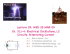### Worksheet 12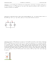### The Series RLC Circuit: Group Worksheet Intro Part 1: Resistive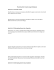### 24- capacitors: capacitance, combination (equivalent capacity), dielectric materials, Tomorrow – Quiz 2: Thursday/Friday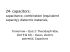### Elektrostatika: Hukum Coulomb### PHYS 212 – MT2 Summer 2012 Sample 3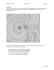### Elantec DC-DC Converter Solution for Virtex FPGAs How to use the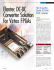### The 6LE8 One Tube Broadcaster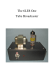### Thrust Force Analysis of a Rotating Ionocraft Under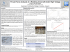### PHYS 212 – MT2 Summer 2012 Sample 2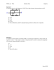### Homework 6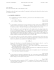### Time dependent circuits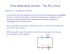### UK-electronic ©2007/14 Assembly manual for the Kit Overdrive TS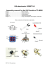### TONE SOURCE TSi2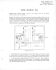### LC102 brochure-5883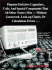### ZENN Motor Company Announces Results Of Independent Testing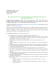### Physics 30 Circuits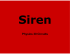### Modifying the Yaesu FT-847 External 22.625 MHz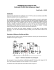### (A) Find the current in the circuit.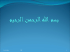### LF198/LF298/LF398, LF198A/LF398A Monolithic Sample-and-Hold Circuits LF198/LF298/LF398, LF198A/LF398A